Скачать презентацию The Fault-Tolerant Group Steiner Problem Rohit Khandekar IBM

3dc988d47340f3cf05d4b5441173082e.ppt

• Количество слайдов: 22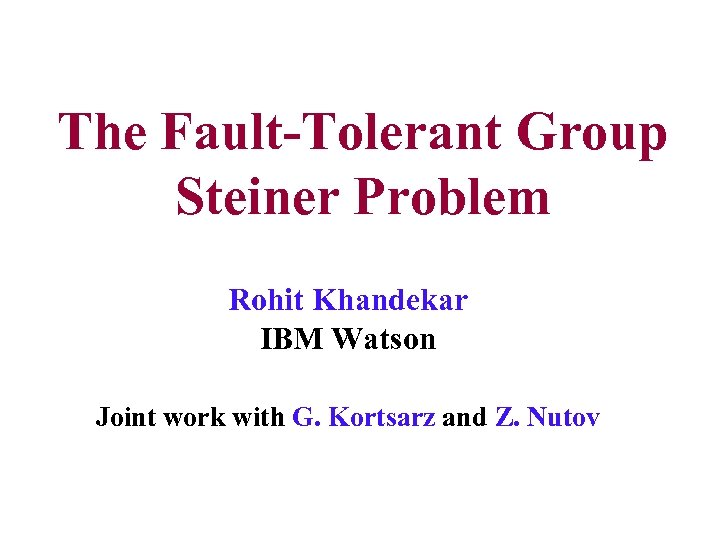The Fault-Tolerant Group Steiner Problem Rohit Khandekar IBM Watson Joint work with G. Kortsarz and Z. Nutov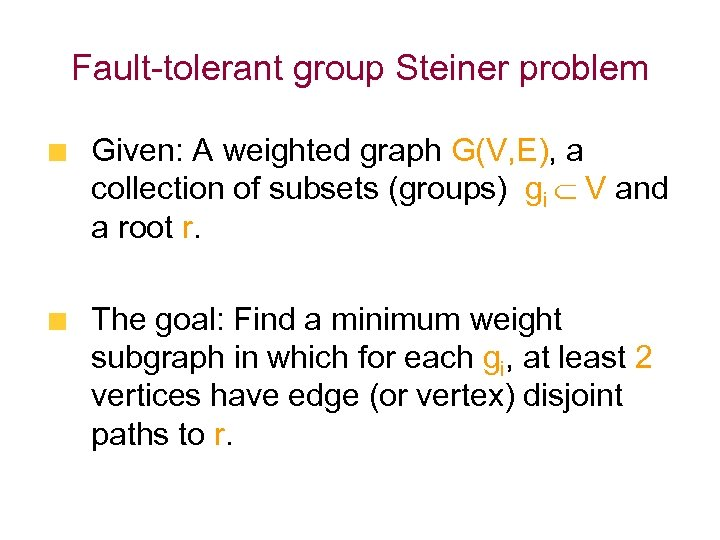Fault-tolerant group Steiner problem Given: A weighted graph G(V, E), a collection of subsets (groups) gi V and a root r. The goal: Find a minimum weight subgraph in which for each gi, at least 2 vertices have edge (or vertex) disjoint paths to r.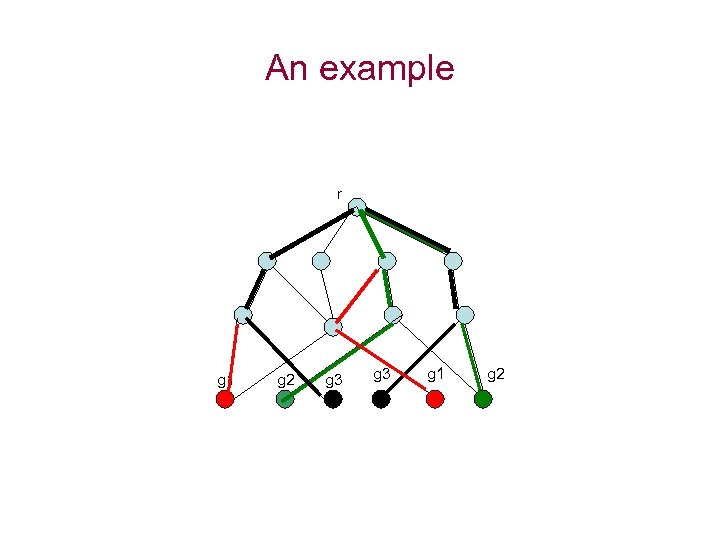An example r g 1 g 2 g 3 g 1 g 2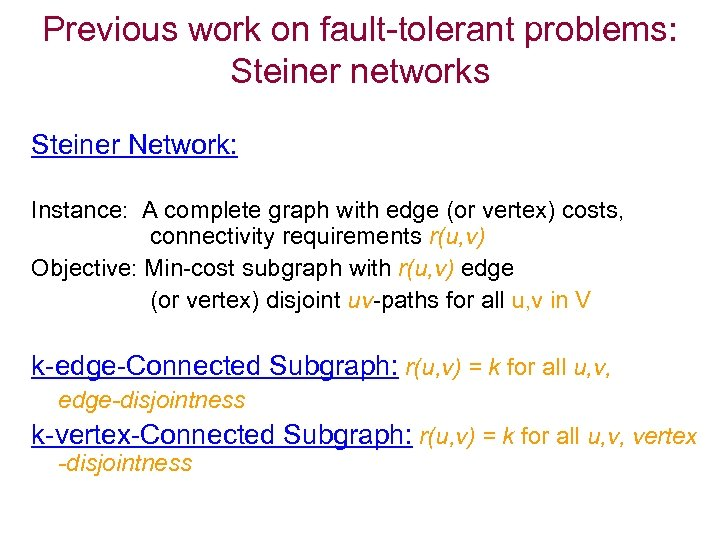Previous work on fault-tolerant problems: Steiner networks Steiner Network: Instance: A complete graph with edge (or vertex) costs, connectivity requirements r(u, v) Objective: Min-cost subgraph with r(u, v) edge (or vertex) disjoint uv-paths for all u, v in V k-edge-Connected Subgraph: r(u, v) = k for all u, v, edge-disjointness k-vertex-Connected Subgraph: r(u, v) = k for all u, v, vertex -disjointness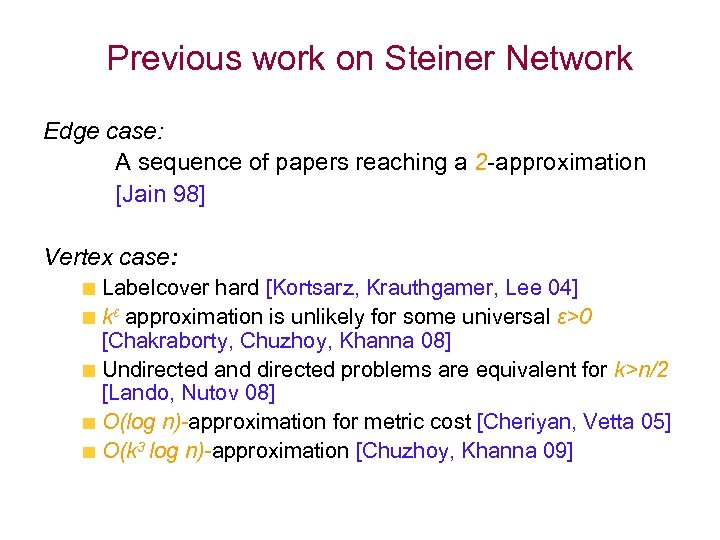Previous work on Steiner Network Edge case: A sequence of papers reaching a 2 -approximation [Jain 98] Vertex case: Labelcover hard [Kortsarz, Krauthgamer, Lee 04] kε approximation is unlikely for some universal ε>0 [Chakraborty, Chuzhoy, Khanna 08] Undirected and directed problems are equivalent for k>n/2 [Lando, Nutov 08] O(log n)-approximation for metric cost [Cheriyan, Vetta 05] O(k 3 log n)-approximation [Chuzhoy, Khanna 09]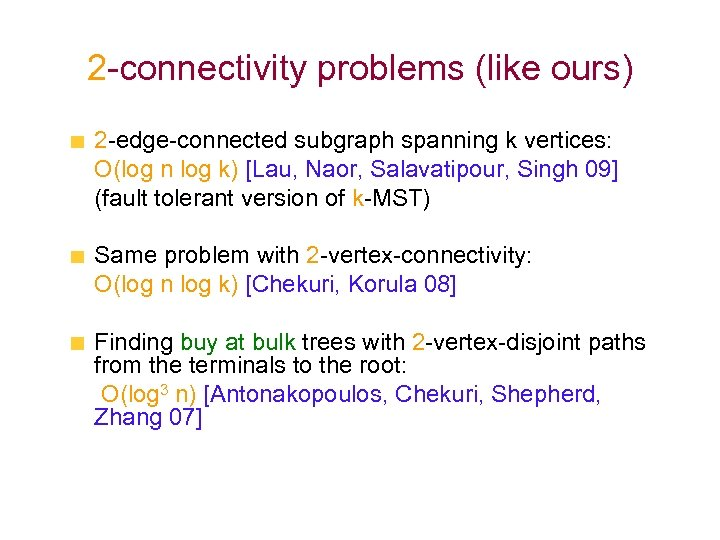2 -connectivity problems (like ours) 2 -edge-connected subgraph spanning k vertices: O(log n log k) [Lau, Naor, Salavatipour, Singh 09] (fault tolerant version of k-MST) Same problem with 2 -vertex-connectivity: O(log n log k) [Chekuri, Korula 08] Finding buy at bulk trees with 2 -vertex-disjoint paths from the terminals to the root: O(log 3 n) [Antonakopoulos, Chekuri, Shepherd, Zhang 07]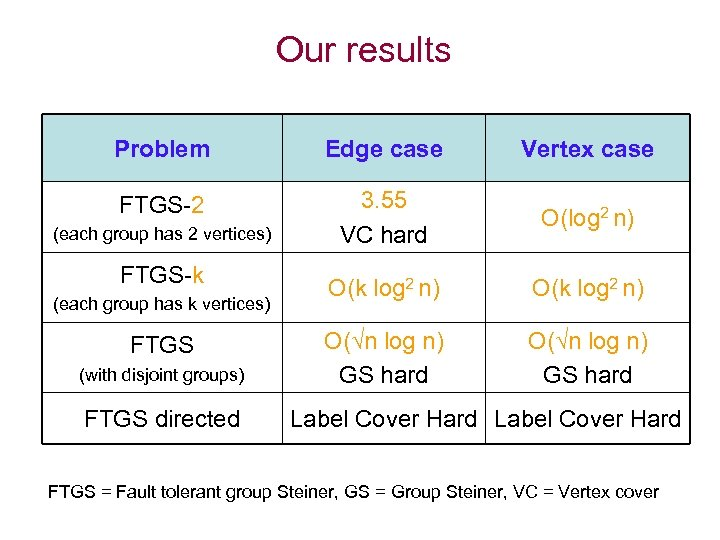Our results Problem Edge case Vertex case FTGS-2 3. 55 VC hard O(log 2 n) O(k log 2 n) O( n log n) GS hard (each group has 2 vertices) FTGS-k (each group has k vertices) FTGS (with disjoint groups) FTGS directed Label Cover Hard FTGS = Fault tolerant group Steiner, GS = Group Steiner, VC = Vertex cover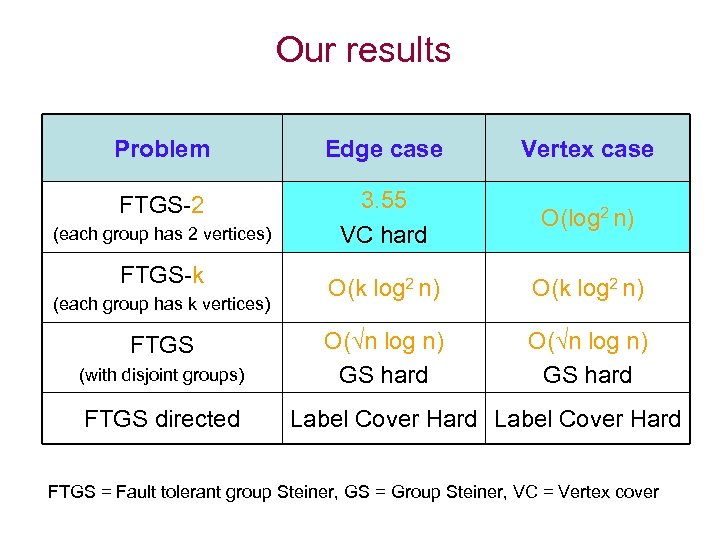Our results Problem Edge case Vertex case FTGS-2 3. 55 VC hard O(log 2 n) O(k log 2 n) O( n log n) GS hard (each group has 2 vertices) FTGS-k (each group has k vertices) FTGS (with disjoint groups) FTGS directed Label Cover Hard FTGS = Fault tolerant group Steiner, GS = Group Steiner, VC = Vertex cover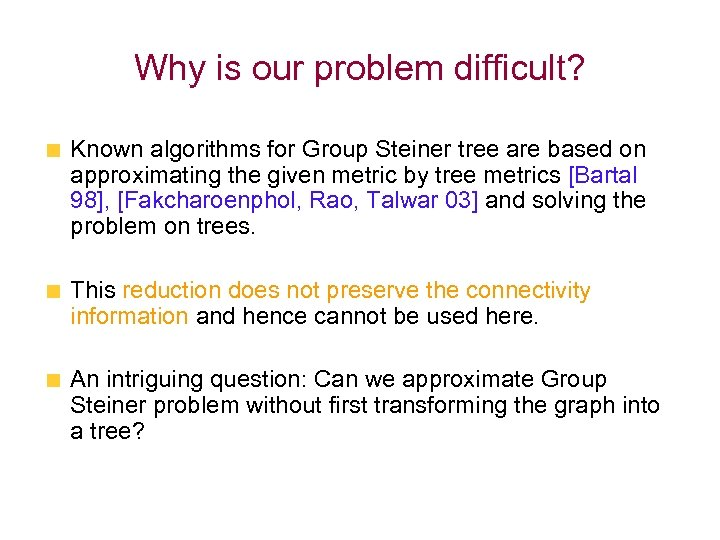Why is our problem difficult? Known algorithms for Group Steiner tree are based on approximating the given metric by tree metrics [Bartal 98], [Fakcharoenphol, Rao, Talwar 03] and solving the problem on trees. This reduction does not preserve the connectivity information and hence cannot be used here. An intriguing question: Can we approximate Group Steiner problem without first transforming the graph into a tree?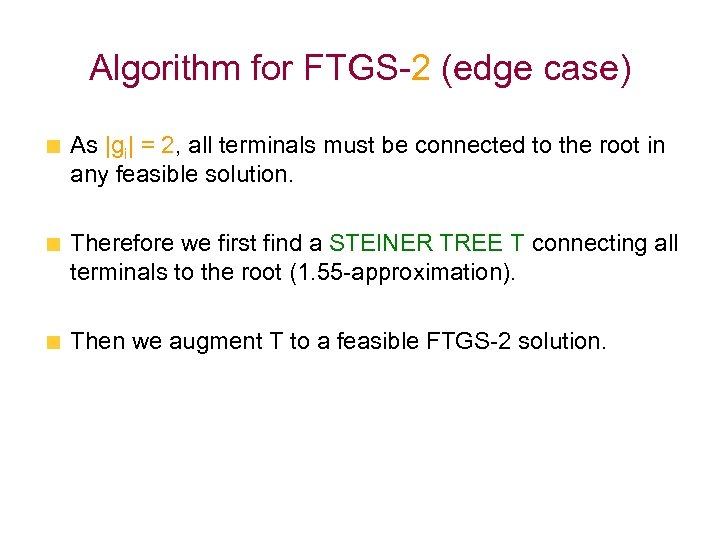Algorithm for FTGS-2 (edge case) As |gi| = 2, all terminals must be connected to the root in any feasible solution. Therefore we first find a STEINER TREE T connecting all terminals to the root (1. 55 -approximation). Then we augment T to a feasible FTGS-2 solution.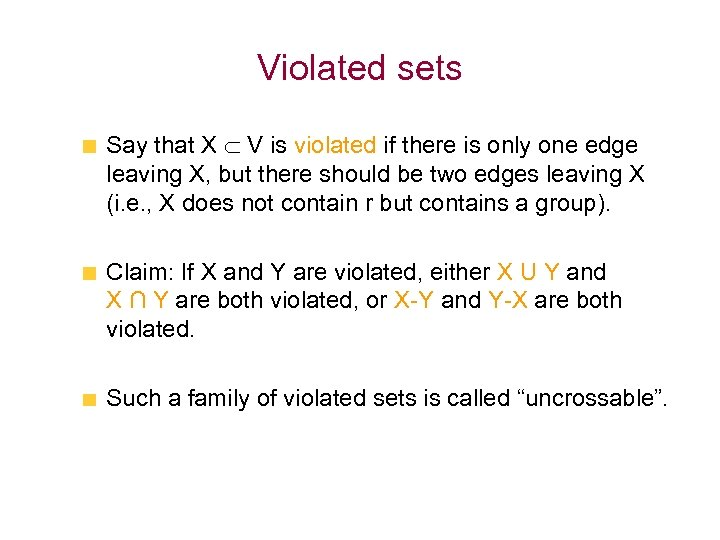Violated sets Say that X V is violated if there is only one edge leaving X, but there should be two edges leaving X (i. e. , X does not contain r but contains a group). Claim: If X and Y are violated, either X U Y and X ∩ Y are both violated, or X-Y and Y-X are both violated. Such a family of violated sets is called “uncrossable”.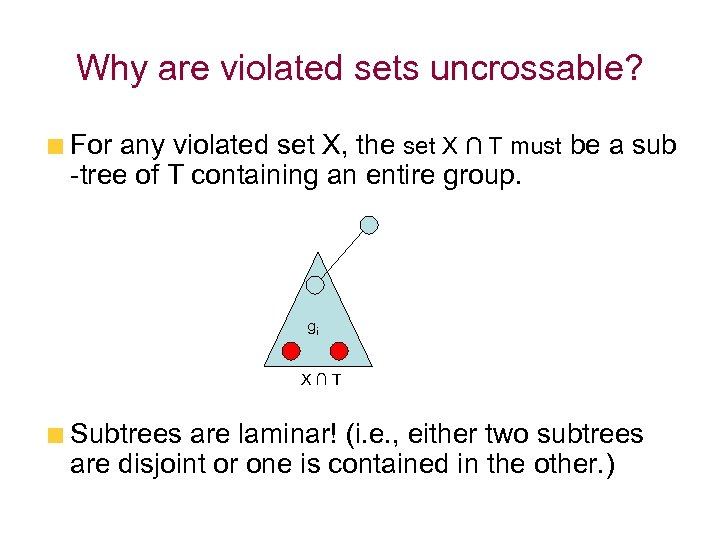Why are violated sets uncrossable? For any violated set X, the set X ∩ T must be a sub -tree of T containing an entire group. gi X∩T Subtrees are laminar! (i. e. , either two subtrees are disjoint or one is contained in the other. )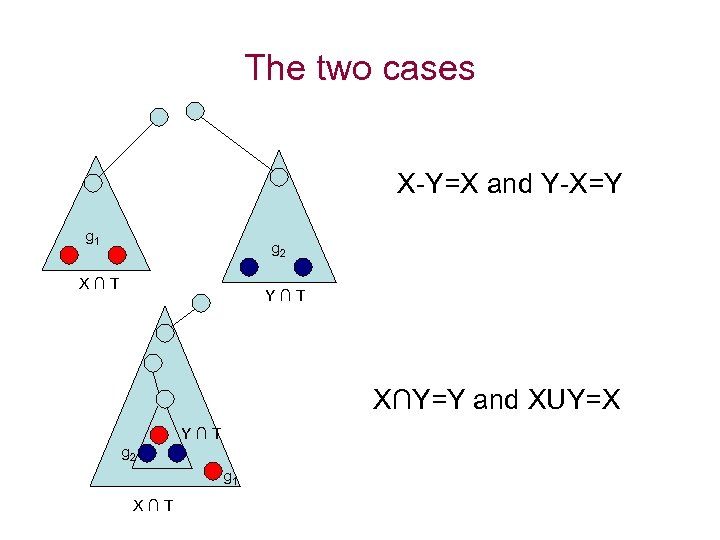The two cases X-Y=X and Y-X=Y g 1 g 2 X∩T Y∩T X∩Y=Y and XUY=X Y∩T g 2 g 1 X∩T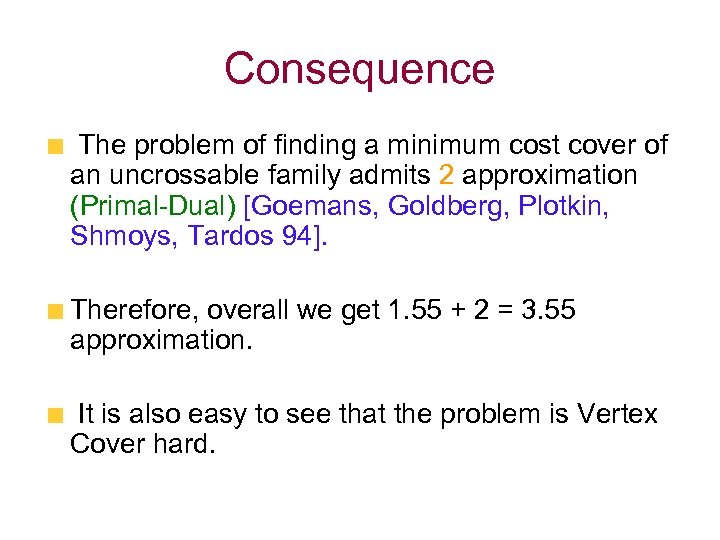Consequence The problem of finding a minimum cost cover of an uncrossable family admits 2 approximation (Primal-Dual) [Goemans, Goldberg, Plotkin, Shmoys, Tardos 94]. Therefore, overall we get 1. 55 + 2 = 3. 55 approximation. It is also easy to see that the problem is Vertex Cover hard.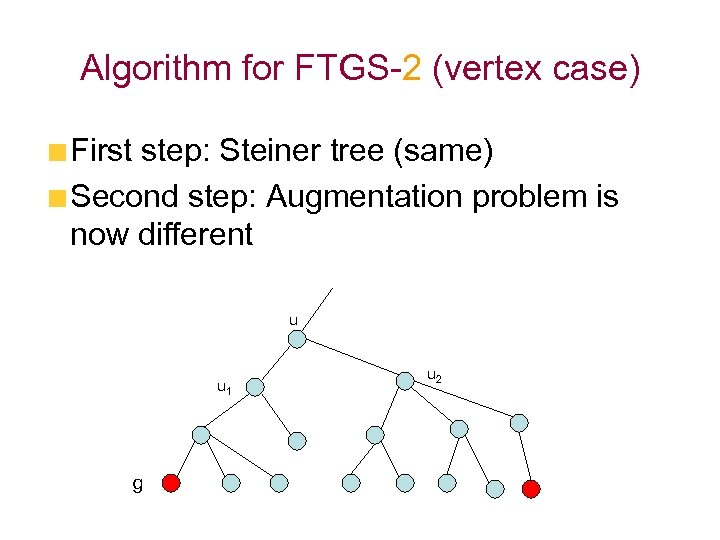Algorithm for FTGS-2 (vertex case) First step: Steiner tree (same) Second step: Augmentation problem is now different u u 1 g u 2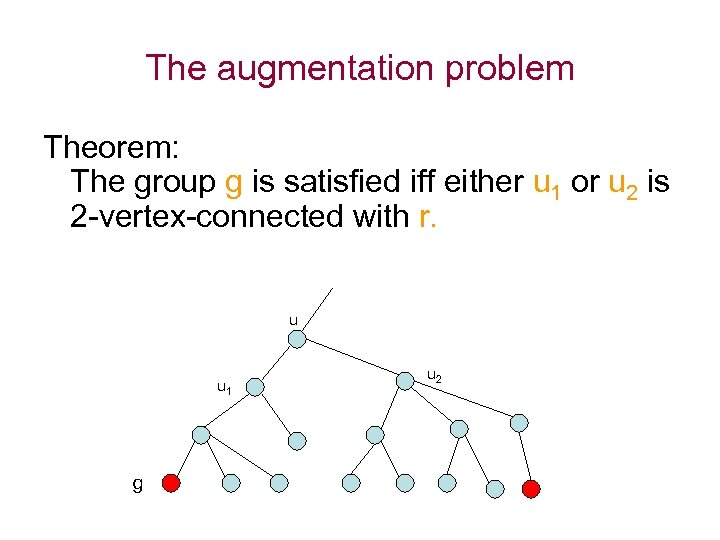The augmentation problem Theorem: The group g is satisfied iff either u 1 or u 2 is 2 -vertex-connected with r. u u 1 g u 2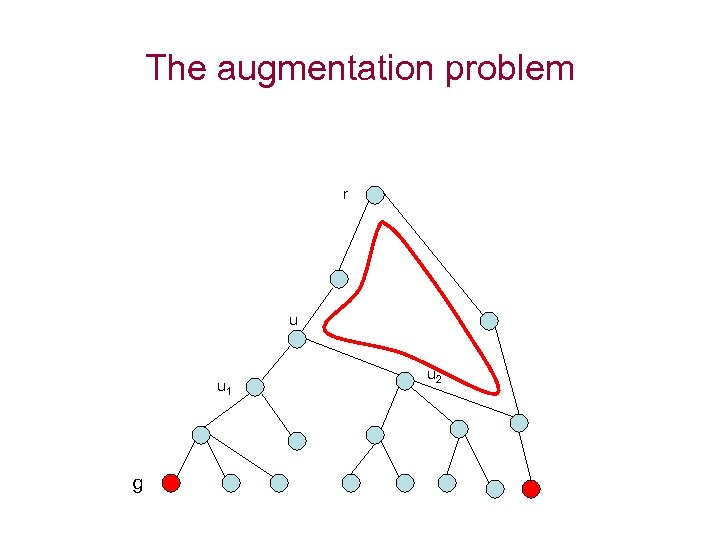The augmentation problem r u u 1 g u 2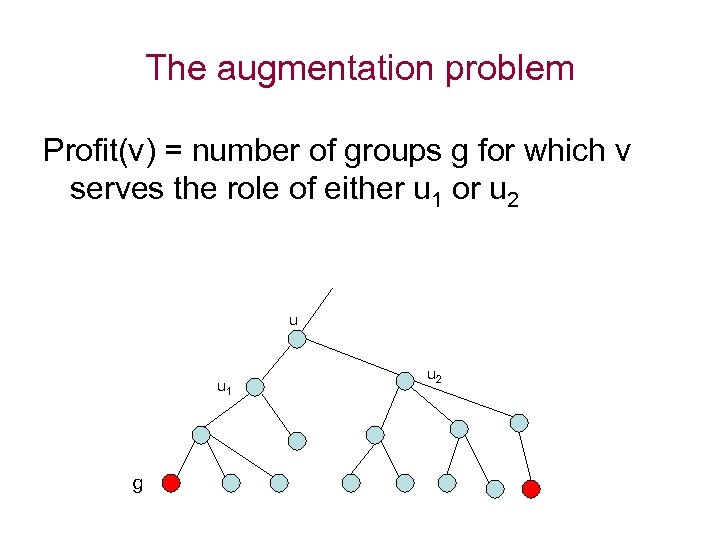The augmentation problem Profit(v) = number of groups g for which v serves the role of either u 1 or u 2 u u 1 g u 2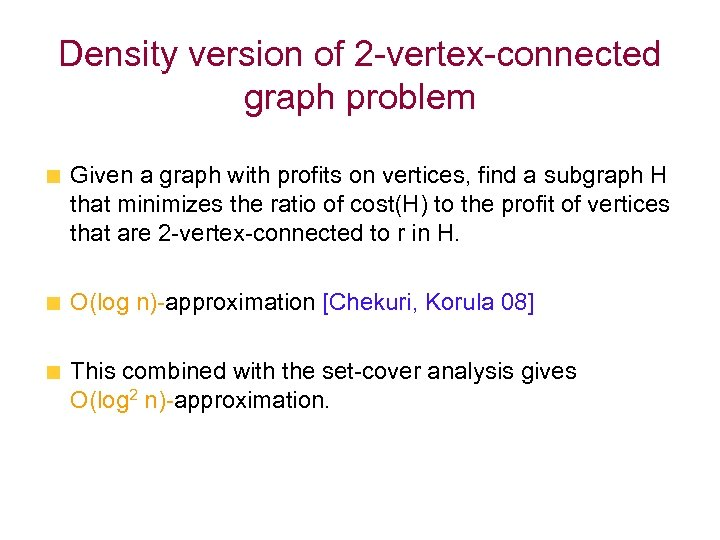Density version of 2 -vertex-connected graph problem Given a graph with profits on vertices, find a subgraph H that minimizes the ratio of cost(H) to the profit of vertices that are 2 -vertex-connected to r in H. O(log n)-approximation [Chekuri, Korula 08] This combined with the set-cover analysis gives O(log 2 n)-approximation.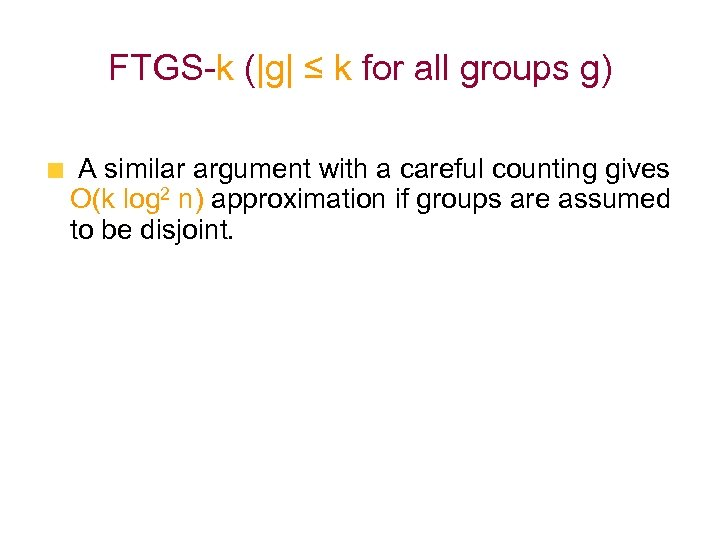FTGS-k (|g| ≤ k for all groups g) A similar argument with a careful counting gives O(k log 2 n) approximation if groups are assumed to be disjoint.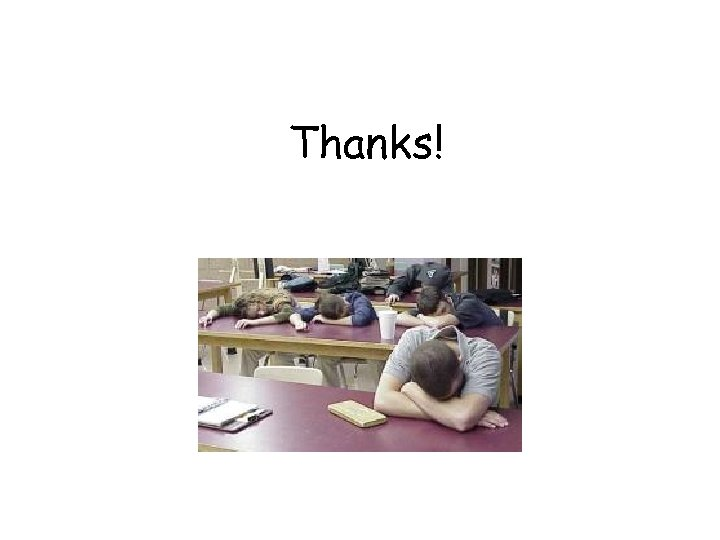Thanks!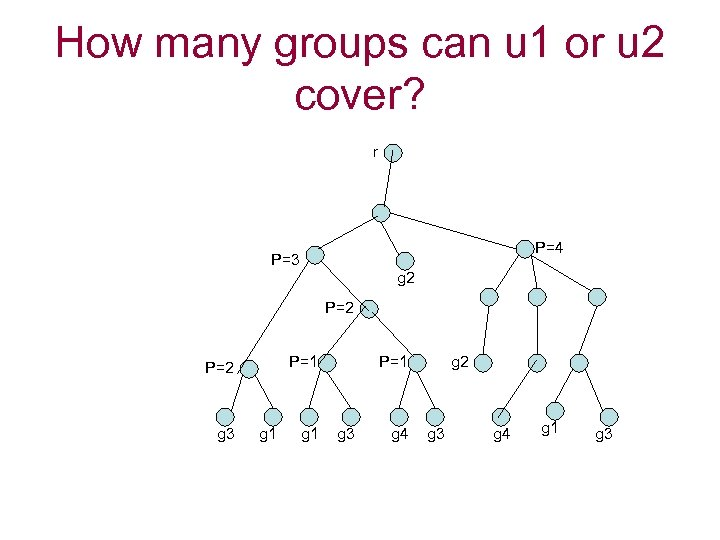How many groups can u 1 or u 2 cover? r P=4 P=3 g 2 P=1 P=2 g 3 g 1 P=1 g 3 g 4 g 2 g 3 g 4 g 1 g 3